# Graphical Analysis - Does a “More Democratic” Society lead to an improvement in the “quality of life”?

As a part of the Data Management and Visualisation course on Coursera, I shall be working on the following hypothesis and trying to test it from the GapMinder Dataset:

What effect does the “level of democracy” in a country have on the “quality of life” of the country’s citizens?

I carried out a brief extrapolatory analysis of the dataset to figure out the distribution of the main variables that I shall be using to test my hypothesis. In short, I would be measuring the correlation between the “polityscore” (a numeric value from -10 to 10 that measures how democratic a country is)variable and the following variables in the dataset

• incomeperperson(2010 GDP per capita in constant US\$ 2000)

• alconconsumption(2008 alcohol consumption per adult)

• breastcancerper100TH(breast cancer new cases per 10,000)

• employrate(2007 total emloyees over 15+)

• HIVrate(2009 estimated HIV prevalence)

• lifeexpectancy(2011 life expectancy at birth)

• urbanrate(Urban population)

The following code block was used for converting the required variables to the proper format in order to carry out graphical analysis of them.

``````import pandas as pd
import numpy as np
data = pd.read_csv(‘gapminder.csv’, low_memory=False)
#setting variables you will be working with to numeric
data[‘incomeperperson’] = data[‘incomeperperson’].convert_objects(convert_numeric=True)
data[‘alcconsumption’] = data[‘alcconsumption’].convert_objects(convert_numeric=True)
data[‘breastcancerper100th’] = data[‘breastcancerper100th’].convert_objects(convert_numeric=True)
data[‘employrate’] = data[‘employrate’].convert_objects(convert_numeric=True)
data[‘hivrate’] = data[‘hivrate’].convert_objects(convert_numeric=True)
data[‘lifeexpectancy’] = data[‘lifeexpectancy’].convert_objects(convert_numeric=True)
data[‘urbanrate’] = data[‘urbanrate’].convert_objects(convert_numeric=True)
data[‘polityscore’] = data[‘polityscore’].convert_objects(convert_numeric=True)
#select data subset that does not have any missing polity scores
subset = data[np.isfinite(data[‘polityscore’])]
subset = subset[np.isfinite(subset[‘incomeperperson’])]
subset = subset[np.isfinite(subset[‘alcconsumption’])]
subset = subset[np.isfinite(subset[‘breastcancerper100th’])]
subset = subset[np.isfinite(subset[‘employrate’])]
subset = subset[np.isfinite(subset[‘hivrate’])]
subset = subset[np.isfinite(subset[‘lifeexpectancy’])]
subset = subset[np.isfinite(subset[‘urbanrate’])]
``````

The following were the histograms generated for each variable in question, along with a brief description of their spread that could be observed.

``````subset[‘lifeexpectancy’].hist(bins=50)
``````

The above is a histogram of the life expectancy values in the dataset. The values have a positive skew to them, although the values are kind of evenly distributed across the age ranges. The average life expectancy of the dataset hovers around the 70 years mark.

``````subset[‘incomeperperson’].hist(bins=50)
``````

The values for the income distribution across the dataset are highly negatively skewed. The majority of the people in the dataset have an average income of between \$ 0 to \$5000.

``````subset[‘alcconsumption’].hist(bins=50)
``````

The values of alcohol consumption are again very negatively skewed. A major reason for this might be the fact that in most of the developing countries, the rates of alcohol consumption are not very well recorded. Therefore, even though these countries might be suffering from alcoholism coupled with poor economic conditions, these stats are not necessarily reflected in the data.

``````subset[‘breastcancerper100th’].hist(bins=50)
``````

Although the rates of breast cancer are also negatively skewed, there is a huge occurrence of 20–40 cases of breast cancer per 10,000 population. Also, in the graph, there can be observed a subset of countries which have very high breast cancer rates, around 80–95 rates per 10,000 people.

``````subset[‘employrate’].hist(bins=50)
``````

The rate of employment can be said to be somewhat evenly distributed. The median rate of employment is between 55 to 65 .

``````subset[‘hivrate’].hist(bins=50)
``````

The rates of HIV are very negatively skewed. However, the distribution of this does not do justice to the fact that a lot of countries, the rate of HIV, although being “low” on an absolute scale, is still problematic high and needs to be fixed.

``````subset[‘urbanrate’].hist(bins=50)
``````

The data for the urban rates in the countries is very evenly distributed, with a slight positive skew. The average urban rate lies somewhere between 50–70 people per 1000 in the dataset.

Now, for the more interesting analysis.

I decided to plot boxplots for all of the predictor variables against the the polityscores. This would give a good picture of how exactly each variable changed with how “democratic” or “autocratic” a country was.

The following code was used to draw the different boxplots.

``````subset.boxplot(column=’incomeperperson’,by=’polityscore’)
subset.boxplot(column=’alcconsumption’,by=’polityscore’)
subset.boxplot(column=’breastcancerper100th’,by=’polityscore’)
subset.boxplot(column=’employrate’,by=’polityscore’)
subset.boxplot(column=’hivrate’,by=’polityscore’)
subset.boxplot(column=’lifeexpectancy’,by=’polityscore’)
subset.boxplot(column=’urbanrate’,by=’polityscore’)
``````

The following are the boxplots that were generated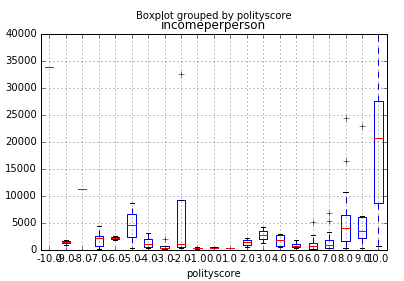Boxplot of Average Income per person against polityscore

There is a strong positive correlation between how “democratic” an economy is and the average annual income level.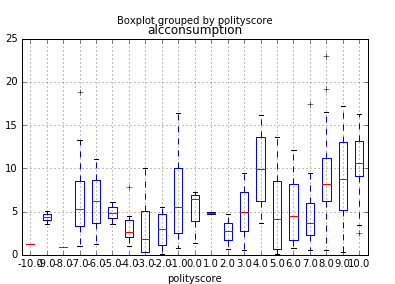Boxplot of Alcohol consumption against polityscore

Surprisingly, the amount of alcohol consumption goes up as an economy becomes more ‘democratic’. However, this increase might only be due to a country becoming more “open”. There is not much data to infer whether this increased alcohol consumption actually leads to any detrimental effects in the economy.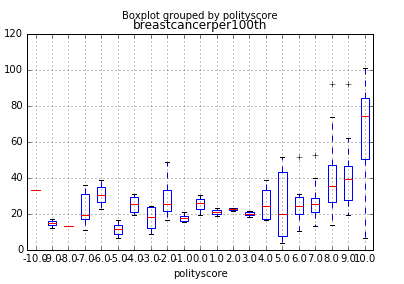Boxplot of Rates of Breast Cancer cases per 1000 people against polityscore

The rates of breast cancer rise up as the economy becomes more “open”. This is a surprising result, since one would believe that the general level of health and the prevalence of diseases might go down as a country becomes more democratic. However, a possible explanation of this trend might be that a more ‘open’ society leads to more cases of breast cancer being reported, since better healthcare leads to more women being tested for it.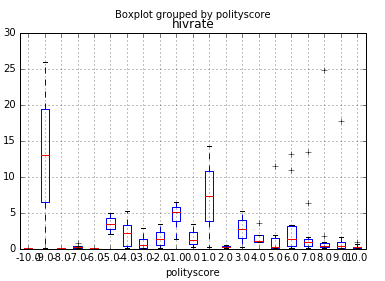Boxplot of HIV rates against polityscore

There is a strong negative correlation between the rates of HIV in a country and how “democratic” it is, which points to an improvement in the healthcare of a country as it becomes more ‘democratic’.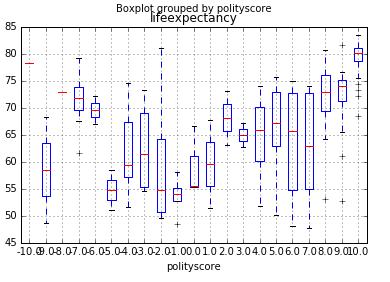Boxplot of life expectancy against polityscore

There is a positive correlation between the life expectancy of a country and its level of ‘democracy’.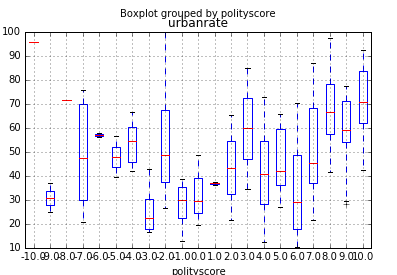Boxplot of urban rate against polityscore

The rate of urbanisation in a country is strongly positively correlated with how “democratic” it is.

This post was originally published on Medium